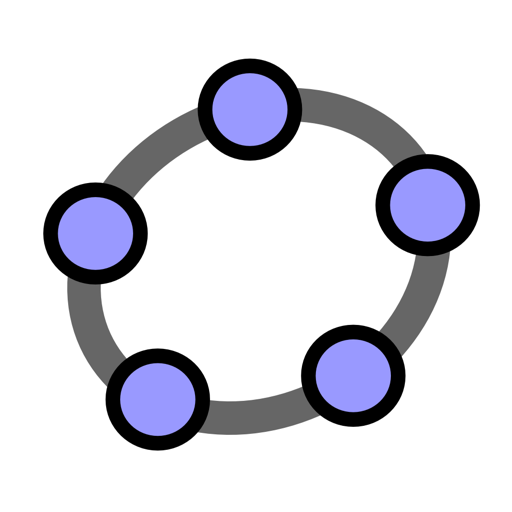# GeoGebra Math Apps for Mac

GeoGebra Math Apps (was GeoGebra) is a dynamic mathematics software for all levels of education that joins arithmetic, geometry, algebra, and calculus. It offers multiple representations of objects in its graphics, algebra, and spreadsheet views that are all dynamically linked.

While other interactive software (e.g., Cabri Geometry, Geometer's Sketchpad) focus on dynamic manipulations of geometrical objects, the idea behind GeoGebra is to connect geometric, algebraic, and numeric representations in an interactive way. You can do constructions with points, vectors, lines, and conic sections, as well as functions, and change them dynamically afterwards. Furthermore, GeoGebra allows you to directly enter and manipulate equations and coordinates. Thus you can easily plot functions, work with sliders to investigate parameters, find symbolic derivatives, and use powerful commands like Root or Sequence.

v6.0.536版本新功能

Version 6.0.536:

• Classic 5: fix for large tool icons
• Corner(-1,13) returns scales for x, y, z axes
• improvement for f(x)=(3/2)^x, f(-1)
• GeoGebra 6: make sure more decimal places (minimum 5) are used when editing an expression
• Editor: fix for ÷ immediately after log₁₀(x) and for eg 3|x|
• LaTeX: fixes for eg v_{1}'^{2} and @{hspace{3.7 mm}}
• Geometry: "Fit to Zoom" preserves aspect ratio
• new default labels for equations eq1, eg2, ...
• CAS View: fix for eg Vector[(x,y,z)] = Vector[(1,2,3)]

Screenshots